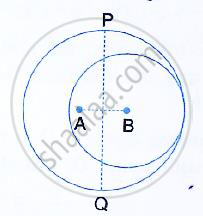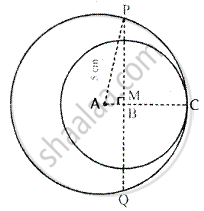Share

Books Shortlist

# The Given Figure Shows Two Circles with Centres a and B; and Radii 5 Cm and 3 Cm Respectively, Touching Each Other Internally. If the Perpendicular Bisector of Ab Meets the Bigger Circle in P and Q, - ICSE Class 10 - Mathematics

ConceptChord Properties - the Perpendicular to a Chord from the Center Bisects the Chord (Without Proof)

#### Question

The given figure shows two circles with centres A and B; and radii 5 cm and 3 cm respectively, touching each other internally. If the perpendicular bisector of AB meets the bigger circle in P and Q, find the length of PQ.#### SolutionJoin AP and produce AB to meet the bigger circle at C.
AB = AC – BC = 5 cm – 3 cm = 2 cm But, M is the mid – point of AB.
∴ AM = 2/2 = 1cm
Now in right ∆APM,

AP^2 = MP^2 + AM^2     [Pythagoras Theorem]
⇒  (5)^2 = MP^2 +1
⇒ 25 = MP^2 1
⇒  MP^2 = 25 -1

⇒ MP^2 = 24

⇒  MP =sqrt24 =sqrt(4 xx 6 )= 2sqrt 6 cm

∴ PQ = 2MP = 2xx 2sqrt 6 = 4sqrt 6 cm
⇒  PQ = 4 xx 2.45 = 9.8 cm

Is there an error in this question or solution?

#### Video TutorialsVIEW ALL 

Solution The Given Figure Shows Two Circles with Centres a and B; and Radii 5 Cm and 3 Cm Respectively, Touching Each Other Internally. If the Perpendicular Bisector of Ab Meets the Bigger Circle in P and Q, Concept: Chord Properties - the Perpendicular to a Chord from the Center Bisects the Chord (Without Proof).
S